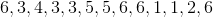# ISEE Lower Level Math : How to identify the parts of a list

## Example Questions

### Example Question #21 : How To Identify The Parts Of A List

For the past 6 days, John has been running, adding a mile to each day. Below are the miles that he ran, per day: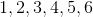What is the median?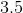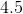Explanation:

The median is the middle number of a set when the numbers in that set are organized from smallest to largest.

When there is an even amount of numbers in a set, meaning that there are 2 middle numbers, the average of those numbers is taken.Here, 3 and 4 are the middle numbers. The median is their average, which would be 3.5.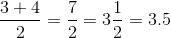### Example Question #742 : Isee Lower Level (Grades 5 6) Mathematics Achievement

As part of her new workout routine, Tracy has been swimming. Below are the number of laps she has swam on a daily basis: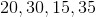What is the mean number of laps?Explanation:

In order to find the mean, the numbers of a set must be added together and then divided by how many numbers are in that set.

To find the mean, the following equation would be used: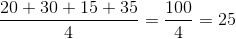The mean is 25.

### Example Question #743 : Isee Lower Level (Grades 5 6) Mathematics Achievement

What is the median of the set?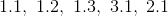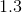None of these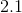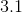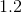Explanation:

In order to find the median of a set, it must first be ordered from smallest to largest.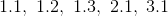Given that there are 5 numbers in this set, the third number is the median. Here, the third number is 1.3, making it the median.

### Example Question #744 : Isee Lower Level (Grades 5 6) Mathematics Achievement

What best describes the shared trait of the numbers in this set?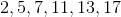Odd

Multiples of 3

Prime

Squares

None of these

Prime

Explanation:

Each of the numbers in this set is divisible only by itself and the number 1; therefore, each of the numbers in this set is prime.

While most of the numbers are odd, the number 2 is even, and so "odd" is not the correct answer.

### Example Question #211 : Data Analysis And Probability

Beth has been keeping track of the lowest temperatures reached each day for the past week. The lowest temperatures, in degrees Farenheit, are: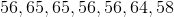What is the mode?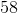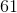Explanation:

The mode is the number that occurs most often in a set.In this set, the temperature of 56 degrees occurs the most often (three times).

### Example Question #212 : Data Analysis And Probability

Jennifer sells textbooks online. She has sold books for the following prices: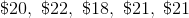What is the median price at which she sells books?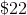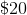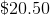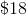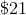Explanation:

The median is the number that is in the middle of a set when organized from smallest to largest.

To find the median of the set, first organize the numbers from smallest to largest.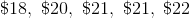The middle number is 21, which makes it the median.

### Example Question #213 : Data Analysis And Probability

What is the median of set below?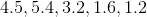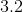Explanation:

In order to find the median of a set, the numbers must be ordered from smallest to largest. The median is thus the middle number.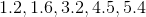Given that the middle number is 3.2, that is the correct answer.

### Example Question #214 : Data Analysis And Probability

What is the mode of the set below?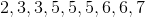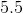Explanation:

The mode is the number that occurs most often in a set. Given that the number 5 appears more often than any other number in the set, it is the mode.

### Example Question #215 : Data Analysis And Probability

During a basketball game, 3 of the players on a team make 4 baskets each, while the other 2 players only make 1 basket each. What is the mean number of baskets that each player made during the game?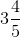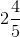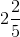Explanation:

The mean is calculated by finding the sum of the numbers in a set, and then dividing that sum by the number of items in the set. Given that 3 of the players made 4 baskets each, while the other 2 players only made only 1 basket each, the equation for finding the mean would look like this (there are 5 players on the team, and so there are 5 items in the set):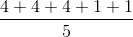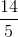### Example Question #216 : Data Analysis And Probability

What is the mode of the set below?